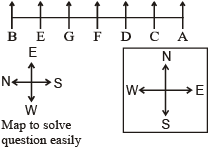# Reasoning Practice Questions for IBPS RRB (Set – 5)

Reasoning Practice Questions for IBPS RRB (Set – 5)
1. Sobha was facing East. She walked 20 metres. Turning left she moved 15 metres and then turning right moved 25 metres. Finally, she turned right and moved 15 metres more. How far is she from her starting point.
a) 25 meters
b) 35 meters
c) 50 meters
d) 45 meters
e) 40 meters
2. Rakesh is on 9th position from upwards and on 38th position from downwards in a class. How many students are in class?
a) 45
b) 46
c) 47
d) 48
e) 49
3. ‘+’ means ‘×’, ‘–’ means ‘÷’, ‘÷’ means ‘+’ and ‘×’ means ‘–’; then what will be the value of the following expression?
540 – 36 + 12 ÷ 75 × 55
a) 255
b) 512
c) 180
d) 235
e) None of these
Directions (4 – 6): ‘P # Q’ means ‘P is not smaller than Q’. ‘P \$ Q’ means ‘P is neither smaller than nor greater than Q’. ‘P @ Q’ means ‘P is neither greater than nor equal to Q’. ‘P * Q’ means ‘P is not greater than Q’. ‘P © Q’ means ‘P is neither smaller than nor equal to Q’. Give Answer:
a) if only conclusion I follows
b) if only conclusion II follows
c) if either conclusion I or II follows
d) if neither conclusion I or II follows
e) if both conclusion I and II follows
4. Statements: B \$ K, K @ D, D # M
Conclusions: I. B \$ M II. B @ M
5. Statements: H @ N, N © W, W # V
Conclusions: I. H @ V II. V @ N
6. Statements: J * D, Q # D, Q @ M
Conclusions: I. Q © J II. Q \$ J
Directions (7 – 10): Study the following information carefully to answer these questions:
A, B, C, D, E, F and G are sitting on a wall facing east.
(i) C is just right of D.
(ii) B is on end point and E is his neighbour.
(iii) G is sitting between E and F.
(iv) D is third from south end.
7. Which of the following is a pair of parsons who is on end points?
a) AE
b) AB
c) FB
d) CB
e) Can’t be determined
8. Which of the following information is not necessary to determine the position of A?
a) i
b) ii
c) iii
d) all information is necessary
e) none of these
9. D is seated between which of the following pairs?
a) CE
b) AC
c) CF
d) AF
e) None of these
10. C want his seat as third from north, he will have to exchange the seat from which person?
a) G
b) F
c) E
d) Can’t be determined
e) None of these
Solutions:
1. D) Shobha turns left after walking 20 metres towards East. Now she walks 15 metres towards North. She turns right towards East again and walks 25 metres further. Finally turning right towards South, she walks 15 metres. The distance moved towards North and towards South is same, i.e., 15 metres. So, Shobha is 20 + 25 metres = 45 metres away from her starting point.2. B) Total students = [Rakesh’s position from upwards + Rakesh’s position from downwards] – 1 = [9 + 38] – 1 = 46
3. E) ? = 540 ÷ 36 × 12 + 75 – 55
or ? = 15 × 12 + 75 – 55
or ? = 180 + 75 – 55 = 200
4. D) B = K …(i)
K < D …(ii) D > M …(iii)
From (i) and (ii), we get D > K = B …(iv)
From (iii) and (iv), no specific relation can be obtained between B and M. Therefore, B = M (Conclusion I) and B < M (Conclusion II) are not necessarily true.
5. B) H < N … (i) N > W …(ii)
W ≥ V …(iii)
From (ii) and (iii), we get N > W ≥ V …(iv)
From (i) and (iv), no specific relation can be obtained between H and V. Hence, H < V (Conclusion I) is not necessarily true. But V < N (Conclusion II) follows from equation (iv).
6. C) J ≤ D …(i)
Q ≥ D …(ii)
Q < M …(iii) Combining (i) and (ii), we get Q ≥ D ≥ J Q > J (Conclusion I) or Q = J (Conclusion II). Hence, either conclusion I or conclusion II is true.
(7 – 10):7. B)   8. D)   9. C)   10. A)